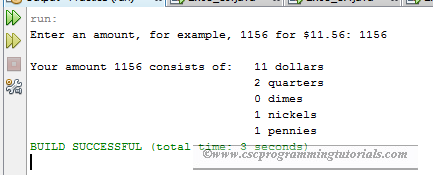# Java Program To Compute Change

The program below is the solution to Liang's Introduction to Java Programming (9th Edition) Chapter 2 Exercise 2.24.

Question: Rewrite Listing 2.10, ComputeChange.java, to fix the possible loss of accuracy when converting a double value to an int value. Enter the input as an integer whose last two digits represent the cents. For example, the input 1156 represents 11 dollars and 56 cents.

```/**
*
* @Author: Aghatise Osazuwa
* Website: www.cscprogrammingtutorials.com
*
* Exercise 2.24 - Financial application: monetary units
*
*/

import java.util.Scanner;

public class ComputeChange {

public static void main(String[] args) {

Scanner input = new Scanner(System.in);
System.out.print("Enter an amount, for example, 1156 for \$11.56: ");
int amount = input.nextInt();
int remainingAmount = amount;
int numberOfOneDollars = remainingAmount / 100;
remainingAmount = remainingAmount % 100;
// Find the number of quarters in the remaining amount
int numberOfQuarters = remainingAmount / 25;
remainingAmount = remainingAmount % 25;
// Find the number of dimes in the remaining amount
int numberOfDimes = remainingAmount / 10;
remainingAmount = remainingAmount % 10;
// Find the number of nickels in the remaining amount
int numberOfNickels = remainingAmount / 5;
remainingAmount = remainingAmount % 5;
// Find the number of pennies in the remaining amount
int numberOfPennies = remainingAmount;
// Display results
System.out.println("\nYour amount " + amount + " consists of: \t"
+ numberOfOneDollars + " dollars\n"
+ "\t\t\t\t" + numberOfQuarters + " quarters\n"
+ "\t\t\t\t" + numberOfDimes + " dimes\n"
+ "\t\t\t\t" + numberOfNickels + " nickels\n"
+ "\t\t\t\t" + numberOfPennies + " pennies");
}
}
```Program Output
Click here to see other solutions to Introduction to Java Programming.